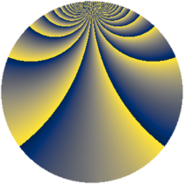# Properties

 Label 2415.2.cgLevel $2415$ Weight $2$ Character orbit 2415.cg Rep. character $\chi_{2415}(64,\cdot)$ Character field $\Q(\zeta_{22})$ Dimension $1440$ Sturm bound $768$

# Related objects

## Defining parameters

 Level: $$N$$ $$=$$ $$2415 = 3 \cdot 5 \cdot 7 \cdot 23$$ Weight: $$k$$ $$=$$ $$2$$ Character orbit: $$[\chi]$$ $$=$$ 2415.cg (of order $$22$$ and degree $$10$$) Character conductor: $$\operatorname{cond}(\chi)$$ $$=$$ $$115$$ Character field: $$\Q(\zeta_{22})$$ Sturm bound: $$768$$

## Dimensions

The following table gives the dimensions of various subspaces of $$M_{2}(2415, [\chi])$$.

Total New Old
Modular forms 3920 1440 2480
Cusp forms 3760 1440 2320
Eisenstein series 160 0 160

## Trace form

 $$1440q + 136q^{4} + 8q^{6} + 144q^{9} + O(q^{10})$$ $$1440q + 136q^{4} + 8q^{6} + 144q^{9} - 8q^{15} - 120q^{16} + 40q^{20} - 8q^{21} - 24q^{24} + 36q^{25} - 80q^{26} + 16q^{29} + 8q^{30} + 32q^{31} + 12q^{34} - 8q^{35} - 136q^{36} + 72q^{39} + 40q^{40} + 216q^{44} + 32q^{46} + 144q^{49} + 56q^{50} - 16q^{51} + 36q^{54} + 84q^{55} + 48q^{56} - 16q^{59} + 32q^{60} + 48q^{61} + 216q^{64} - 8q^{65} + 16q^{66} - 8q^{69} + 8q^{70} - 16q^{71} - 112q^{74} - 16q^{75} + 232q^{76} + 80q^{79} - 144q^{81} + 24q^{84} + 4q^{85} + 832q^{86} - 16q^{89} + 320q^{91} - 176q^{94} - 192q^{95} + 48q^{96} + O(q^{100})$$

## Decomposition of $$S_{2}^{\mathrm{new}}(2415, [\chi])$$ into newform subspaces

The newforms in this space have not yet been added to the LMFDB.

## Decomposition of $$S_{2}^{\mathrm{old}}(2415, [\chi])$$ into lower level spaces

$$S_{2}^{\mathrm{old}}(2415, [\chi]) \cong$$ $$S_{2}^{\mathrm{new}}(115, [\chi])$$$$^{\oplus 4}$$$$\oplus$$$$S_{2}^{\mathrm{new}}(345, [\chi])$$$$^{\oplus 2}$$$$\oplus$$$$S_{2}^{\mathrm{new}}(805, [\chi])$$$$^{\oplus 2}$$